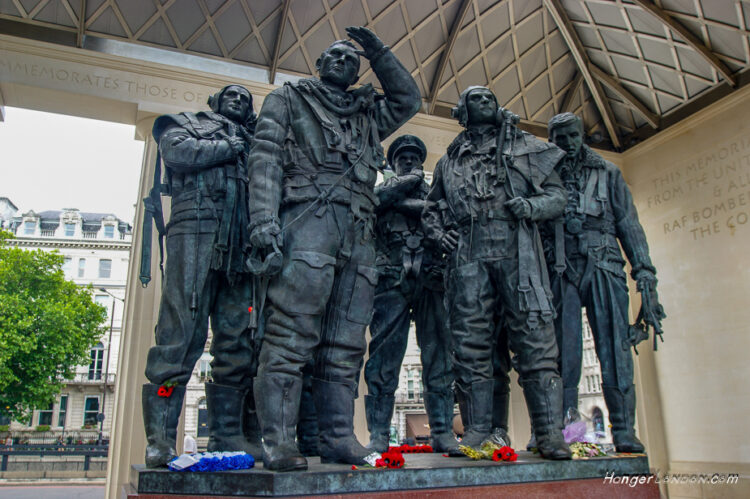# 1940 RAF Bomber Command launched

it’s night attack around Berlin in Germany in retaliation for the attack on London on the 24th August. From the Bomber Command Diaries

Article Rating
Subscribe
Notify of
Inline Feedbacks

## Maths Quiz: Test your basic Maths

/9
10
Created on

Mathematic Quiz - Prime and Composite Numbers

Math quiz helps us to increase our knowledge

1 / 9

is 908 PRIME number or a COMPOSITE number?

2 / 9

is 529 PRIME number or a COMPOSITE number?

3 / 9

is 29 PRIME number or a COMPOSITE number?

4 / 9

is 61 a Prime number or a Composite number?

5 / 9

is 850 PRIME number or a COMPOSITE number?

6 / 9

is 601 PRIME number or a COMPOSITE number?

7 / 9

is 441 PRIME number or a COMPOSITE number?

8 / 9

is 987 PRIME number or a COMPOSITE number?

9 / 9

is 16 PRIME number or a COMPOSITE number?